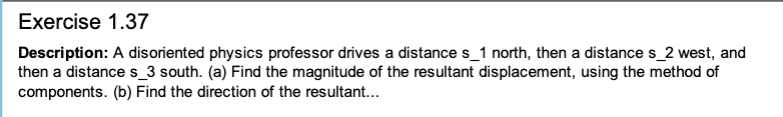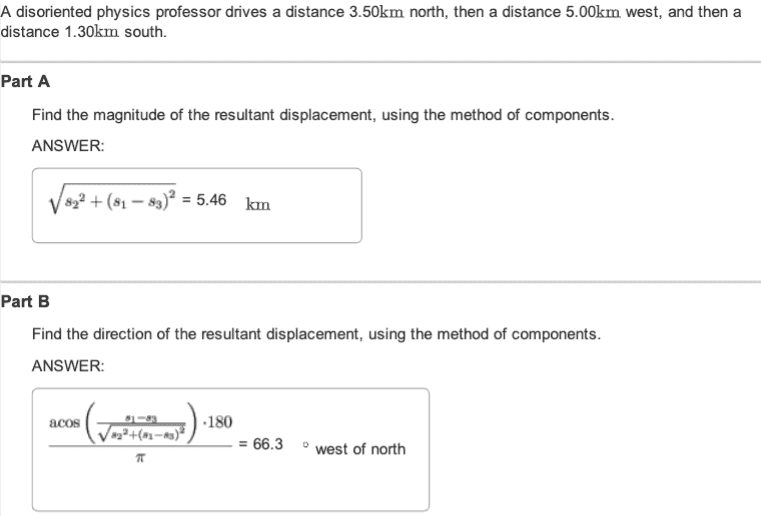# Direction of resulting displacement

• jamiebean

#### jamiebean

Homework Statement
attached below:
Relevant Equations
displacement=final velocity-initial velocityI do not understand part b.
I know that x180/pi changes the value into a degree, but i do not understand what's going on inside the bracket. And how did the equation calculated the direction?

Homework Statement:: attached below:
Relevant Equations:: displacement=final velocity-initial velocity

View attachment 263178View attachment 263179

I do not understand part b.
I know that x180/pi changes the value into a degree, but i do not understand what's going on inside the bracket. And how did the equation calculated the direction?
Suppose the route goes from A north to B, west to C, then south to D.
Draw the diagram.
The angle you want is DAB. That is how much west of north the displacement is.
Now draw a line East from D until it hits the line AB at E. What is the distance AE? You already have the distance AD.
In terms of those, what is the cosine of DAB?

Suppose the route goes from A north to B, west to C, then south to D.
Draw the diagram.
The angle you want is DAB. That is how much west of north the displacement is.
Now draw a line East from D until it hits the line AB at E. What is the distance AE? You already have the distance AD.
In terms of those, what is the cosine of DAB?

thank you! I understand now!•Delta2